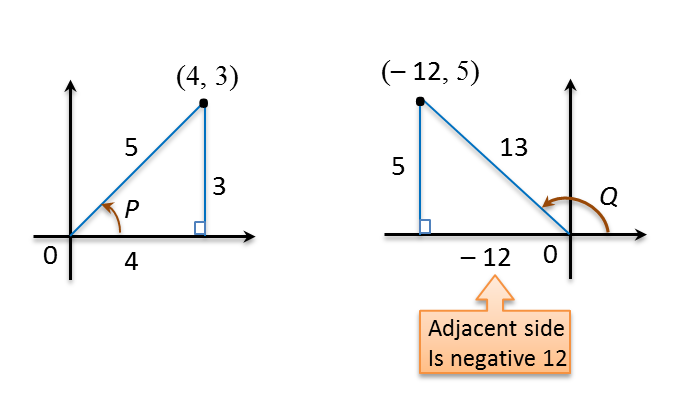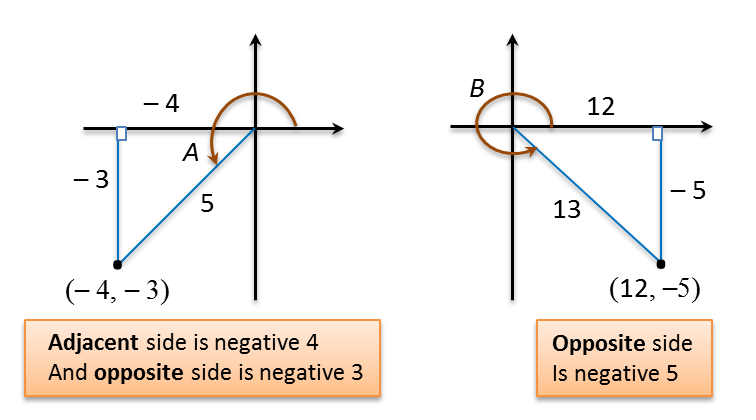# 6.5.1 Proving Trigonometric Identities Using Addition Formula and Double Angle Formulae (Examples)

Example 2:
Prove each of the following trigonometric identities.
$\begin{array}{l}\text{(a)}\frac{1+\mathrm{cos}2x}{\mathrm{sin}2x}=\mathrm{cot}x\\ \text{(b)}\mathrm{cot}A\mathrm{sec}2A=\mathrm{cot}A+\mathrm{tan}2A\\ \text{(c)}\frac{sinx}{1-cosx}=\mathrm{cot}\frac{x}{2}\end{array}$

Solution:
(a)
$\begin{array}{l}LHS\\ =\frac{1+\mathrm{cos}2x}{\mathrm{sin}2x}\\ =\frac{1+\left(2{\mathrm{cos}}^{2}x-1\right)}{2\mathrm{sin}x\mathrm{cos}x}\\ =\frac{\overline{)2}{\mathrm{cos}}^{\overline{)2}}x}{\overline{)2}\mathrm{sin}x\overline{)\mathrm{cos}x}}\\ =\frac{\mathrm{cos}x}{\mathrm{sin}x}\\ =\mathrm{cot}x=RHS\text{(proven)}\end{array}$

(b)
$\begin{array}{l}RHS\\ =\mathrm{cot}A+\mathrm{tan}2A\\ =\frac{\mathrm{cos}A}{\mathrm{sin}A}+\frac{\mathrm{sin}2A}{\mathrm{cos}2A}\\ =\frac{\mathrm{cos}A\mathrm{cos}2A+\mathrm{sin}A\mathrm{sin}2A}{\mathrm{sin}A\mathrm{cos}2A}\\ =\frac{\mathrm{cos}A\left({\mathrm{cos}}^{2}A-{\mathrm{sin}}^{2}A\right)+\mathrm{sin}A\left(2\mathrm{sin}A\mathrm{cos}A\right)}{\mathrm{sin}A\mathrm{cos}2A}\\ =\frac{{\mathrm{cos}}^{3}A-\mathrm{cos}A{\mathrm{sin}}^{2}A+2{\mathrm{sin}}^{2}A\mathrm{cos}A}{\mathrm{sin}A\mathrm{cos}2A}\\ =\frac{{\mathrm{cos}}^{3}A+\mathrm{cos}A{\mathrm{sin}}^{2}A}{\mathrm{sin}A\mathrm{cos}2A}\\ =\frac{\mathrm{cos}A\left({\mathrm{cos}}^{2}A+{\mathrm{sin}}^{2}A\right)}{\mathrm{sin}A\mathrm{cos}2A}\\ =\frac{\mathrm{cos}A}{\mathrm{sin}A\mathrm{cos}2A}←\overline{){\mathrm{sin}}^{2}A+{\mathrm{cos}}^{2}A=1}\\ =\left(\frac{\mathrm{cos}A}{\mathrm{sin}A}\right)\left(\frac{1}{\mathrm{cos}2A}\right)\\ =\mathrm{cot}A\mathrm{sec}2A\end{array}$

(c)
$\begin{array}{l}LHS\\ =\frac{sinx}{1-cosx}\\ =\frac{2sin\frac{x}{2}\mathrm{cos}\frac{x}{2}}{1-\left(1-2si{n}^{2}\frac{x}{2}\right)}←\overline{)\begin{array}{l}\mathrm{sin}x=2sin\frac{x}{2}\mathrm{cos}\frac{x}{2},\\ \mathrm{cos}x=1-2{\mathrm{sin}}^{2}\frac{x}{2}\end{array}}\\ =\frac{\overline{)2}\overline{)sin\frac{x}{2}}\mathrm{cos}\frac{x}{2}}{\overline{)2}si{n}^{\overline{)2}}\frac{x}{2}}=\frac{\mathrm{cos}\frac{x}{2}}{sin\frac{x}{2}}\\ =\mathrm{cot}\frac{x}{2}=RHS\text{(proven)}\end{array}$

Example 3:
(a) Given that  such that P is an acute angle and Q is an obtuse angle, without using tables or a calculator, find the value of cos (P + Q).

(b) Given that  such that A and B are angles in the third and fourth quadrants respectively, without using tables or a calculator, find the value of sin (A
B).

Solution:
(a)$\begin{array}{l}\mathrm{sin}P=\frac{3}{5},\text{}\mathrm{cos}P=\frac{4}{5}\\ \mathrm{sin}Q=\frac{5}{13},\text{}\mathrm{cos}Q=-\frac{12}{13}\\ \\ \mathrm{cos}\left(P+Q\right)\\ =\mathrm{cos}A\mathrm{cos}B-\mathrm{sin}A\mathrm{sin}B\\ =\left(\frac{4}{5}\right)\left(-\frac{12}{13}\right)-\left(\frac{3}{5}\right)\left(\frac{5}{13}\right)\\ =-\frac{48}{65}-\frac{15}{65}\\ =-\frac{63}{65}\end{array}$

(b)$\begin{array}{l}\mathrm{sin}A=-\frac{3}{5},\text{}\mathrm{cos}A=-\frac{4}{5}\\ \mathrm{sin}B=-\frac{5}{13},\text{}\mathrm{cos}B=\frac{12}{13}\\ \\ sin\left(A-B\right)\\ =sinA\mathrm{cos}B-cosA\mathrm{sin}B\\ =\left(-\frac{3}{5}\right)\left(\frac{12}{13}\right)-\left(-\frac{4}{5}\right)\left(-\frac{5}{13}\right)\\ =-\frac{36}{65}-\frac{20}{65}\\ =-\frac{56}{65}\end{array}$